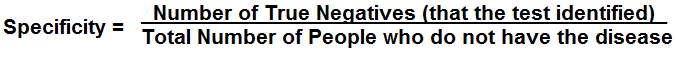﻿ Specificity Calculator ﻿# Specificity CalculatorORThis specificity calculator calculates the specificity of a data sample.

Specificity refers to the test's ability to correctly identify patients who have a do not have certain condition (disease, illness, etc).

In a medical example for a disease, for example, the specificity is the ability of a test to correctly identify patients who do not have a disease.

Therefore, the formula to calculate specificity is, number of true negatives/(number of true negatives + number of false positives).

The number of true negatives refers to the number of people that the test correctly identified did not have the disease (who actually do not have the disease).

The number of false positives refers to the number of people that the test identified that had the disease who actually did not have the disease. Therefore, the test missed this group of people (it got it wrong).

The ratio of the number of true negatives over the number of true negatives plus false positives gives the ratio of how precise, or specific, a test is.

The higher the specificity of a test, the more precise it is in identifying people who do not have a certain condition. Therefore, the more reliable it is.

The specificity of a test shows us how reliable it is or in layman terms, how often the tests accurately tells correctly that a patient does not have a certain disease.

The sensitivity is the exact opposite of specificity. The sensitivity shows how accurately a test can predict that a patient has a disease (true positives rate). While the specificity tells the true negatives rate, the sensitivity gives the true positives rate (how good the test it is at determining that patients have a condition who actually have a condition; in other words, how good it is at not giving false negatives).

To use this calculator, a user simply enters in the number of true negatives, along with the number of false positives, and clicks the "Calculate" button. The resultant specificity (in decimals and percentage) will then be automatically computed and shown.

If you have the number of true negatives and the total amount of the sample (who does not have a disease, for instance), simply take the total amount and subtract the number of true negatives from it; this yields the number of false positives. Then plug these values into the text fields of this calculator.

### Example

A test correctly identified that 41 patients does not have AIDs out of 45 people. The other 4 patients it identified as having AIDs, though they did not. What is the specificity of this test?

Specificity= Number of True Negatives/(Number of True Negatives + Number of False Positives)= 41/(41 + 5)= 40/50= 0.91 or 91%

Related Resources

﻿